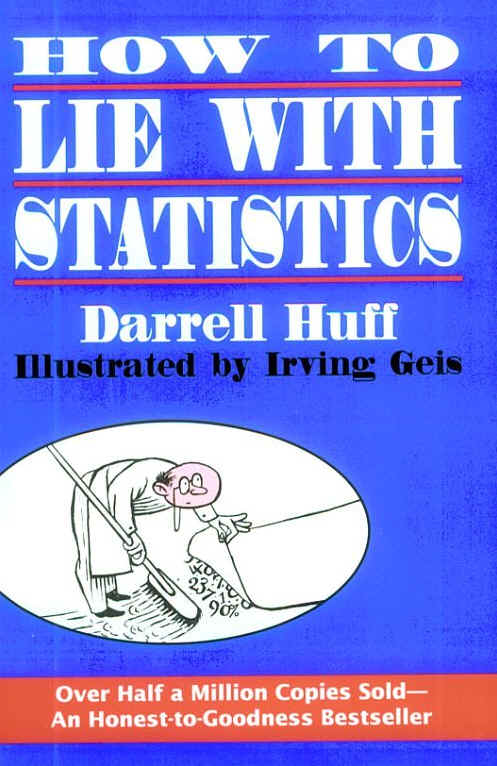# The revised GDP number: pure fictionThe GDP number was revised upwards from 0.6% growth to 0.9% growth. However, what is most interesting is the GDP implicit price deflator. This is the index number the BEA uses to get from nominal GDP to real GDP, the inflation-adjusted number everyone hears on TV and in the news. Nominal GDP was up only 3.5%. So what is your guess about the inflation number the BEA used to get the 0.9% growth number you hear on TV? 3% inflation, 4% inflation?

Try 2.16%. That’s right 2.16%. (The index was 121.31 in Q1 2008, up from 118.75 in Q1 2007. That’s up 2.16%). Now, we all know that there is no way 2.16% is the inflation rate. That’s obviously bogus. So, what if we use the common inflation indicator, the Consumer Price Index (CPI) to deflate the nominal GDP then? What does GDP look like then?

0.38%. That’s getting there. How did I get 0.38%, you ask? I just used the CPI instead of the bogus BEA inflation number.* CPI over this period was 3.10% on a seasonally-adjusted basis.

Another interesting tidbit: in actual fact, CPI ran at an enormous 6.82% annualized rate over those first three months of 2008 without the seasonal adjustments. You say, “of course it was high. Gas and food prices were through the roof.” I agree 100%. So, using the most commonly used inflation index gets you -3.1% GDP, not +0.9%. Now, the annual CPI rate in March 2008 was a still high 4.00% up from March 2007. Even if we use that rate, you get a negative number.

So, what we see here is a sleight of hand. The U.S. government is using a phony 2.16% inflation rate for Q1 2008 to deflate the nominal GDP in order to arrive at 0.9% GDP growth. Actual inflation over that time using the government’s own inflation benchmark was 6.82%. Annual inflation was 4.00% and even seasonally-adjusted quarterly inflation was 3.10%. There’s no way the U.S. economy grew 0.9% in Q1 given this data.

Are the government’s numbers real?

Sources
NIPA Table 1.1.5. Gross Domestic Product, BEA website
NIPA Table 1.1.4. Price Indexes for Gross Domestic Product, BEA website
CPI Historical Data Series, BLS website

*Here’s the math: Take the nominal GDP number for Q4 2007 of \$14,074.2 billion and divide by the seasonally adjusted CPI Index for Dec. 2007 of 211.7. That gets you adj. real GDP for Q4. Then take the nominal GDP number for Q1 2008 of \$14,195.6 billion and divide by the seasonally adjusted CPI Index for Mar. 2008 of 213.3. That gets you adj. real GDP for Q4. Divide Q1 2008 adj. real GDP by Q4 2007 adj. real GDP multiply by 4 to annualize and subtract 1. Presto.